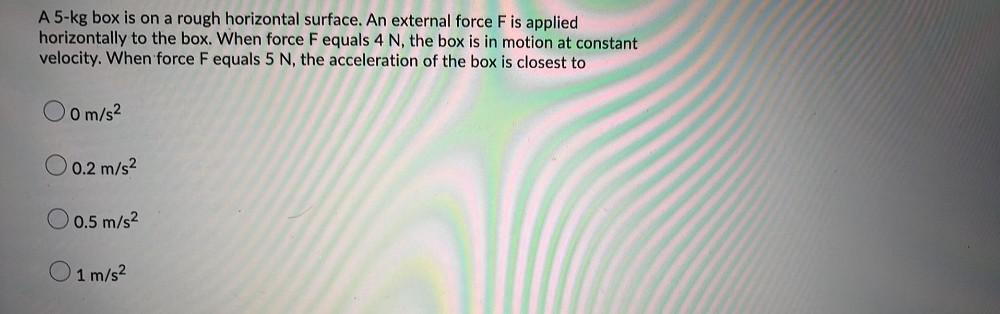Question:

# A 5-kg box is on a rough horizontal surface. An external force F is applied horizontally to the box. When force F equals 4 N, thA 5-kg box is on a rough horizontal surface. An external force F is applied horizontally to the box. When force F equals 4 N, the box is in motion at constant velocity. When force Fequals 5 N, the acceleration of the box is closest to Oom/s² O 0.2 m/s2 0.5 m/s2 O 1 m/s2